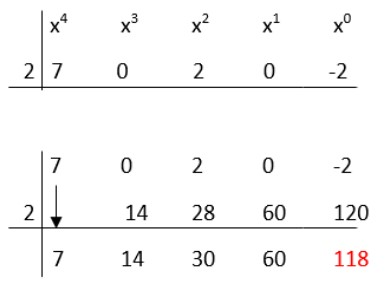# Synthetic Division Calculator

To use a synthetic division calculator, enter a polynomial & a linear factor and hit calculate button

This will be calculated:

Give Us Feedback

## Synthetic Division Calculator

Synthetic division calculator is a tool that performs synthetic division by using the polynomial as a numerator and linear factor as a denominator. The synthetic division calculator gives a step-by-step solution to every problem.

## Definition of Synthetic division

Synthetic division is a method of dividing polynomials by linear factors. It is a very simple technique to divide the polynomial with the linear factor of the form “x-c”. Where “c” is a self-determined constant.

### Formula

The formula of synthetic division is stated as follows.

P(x)/(x-c) = Q(x) + R/(x-c)

Where,

• P(x) = dividend polynomial of any order.
• (x-c) = linear factor of degree 1.
• Q(x) = quotient polynomial formed after applying the synthetic technique.
• R = constant quantity known as the residual or remainder.

### Example of Synthetic Division

Solve the question by synthetic division if,

Numerator polynomial = 7x4+2x2-2

Denominator polynomial = x-2

Solution:

Step 1: Write the given data.

P(x) = 7x4+2x2-2

Linear factor = x-2

P(x)/Linear factor = (7x4+2x2-2)/ (x-2)

Step 2: write the coefficients of the numerator polynomial and write them from greatest to least.

7, 0, 2, 0, −2

Step 3: put the denominator term equal to zero and find the value of the unknown variable.

x2=0

x=2

Step 4: write the above data in the synthetic division and simplify.The quotient is 7x3 + 14x2 + 30x + 60 and the remainder is 118.

Step 5: write the value according to the synthetic division formula.

Q(x) = 7x3 + 14x2 + 30x + 60, R = 118, and c = 2

[7x4+2x2-2/ x-2] = (7x3 + 14x2 + 30x + 60) + [118/ (x-2)]

## Some other examples

Here are a few results of other examples in the table section.

 Numerator Polynomial Denominator Polynomial Quotient Remainder 7x5+2x2-2 x - 5 7x4+35x3+175x2+877x+4385 21923 7x3+4x2+3 x - 4 7x2+32x+128 515 x3+7x2+3 x-2 x2+9x+18 39 x5+7x2+3 x-4 x4+4x3+16x2+71x+284 1139 x6-x3+3 x-2 x5+2x4+4x3+7x2+14x+28 59

### Math Tools